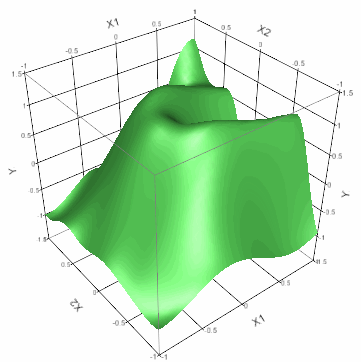Publication date: 11/10/2021

# Gaussian Process

##### Fit Data Using Smoothing Models

Use the Gaussian Process platform to model the relationship between a continuous response and one or more predictors. These types of models are common in computer simulation experiments, such as the output of finite element codes, and they often perfectly interpolate the data. Gaussian processes can deal with these no-error-term models, in which the same input values always results in the same output value.

The Gaussian Process platform fits a spatial correlation model to the data. The correlation of the response between two observations decreases as the values of the independent variables become more distant.

One purpose for using this platform is to obtain a prediction formula that can be used for further analysis and optimization.

Figure 17.1 Gaussian Process Prediction Surface Example